## A pull back toy car accelerates for 1.8 seconds and covers 4 m while speeding up after being released from rest.

Question

A pull back toy car accelerates for 1.8 seconds and covers 4 m while speeding up after being released from rest.

in progress 0
4 months 2021-09-05T12:14:42+00:00 1 Answers 0 views 0

## Answers ( )

Acceleration, a = 2.47 seconds

Explanation:

Given the following data;

Time, t = 1.8 seconds

Distance = 4 meters

Initial velocity = 0 m/s (since it’s starting from rest)

To find the acceleration of the toy car, we would use the second equation of motion;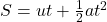Where;

S represents the displacement or height measured in meters.

u represents the initial velocity measured in meters per seconds.

t represents the time measured in seconds.

a represents acceleration measured in meters per seconds square.

Substituting into the equation, we have;

Substituting into the formula, we have;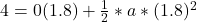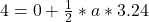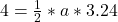Cross multiplying, we have;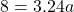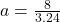Acceleration, a = 2.47 seconds